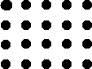Points arranged in array...!

Probability Level 5There is a $4 \times 5$ array of points, each of which is 1 unit away its nearest neighbours . Determine the number of non-degenerate triangles ( i.e. triangles with positive area ) whose vertices are points in the given array.

×